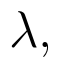Select Page

# 12 Science CBSE Answers for MCQ Maths Integrals in English

12 Science CBSE Answers for MCQ Maths Integrals in English to enable students to get Answers in a narrative video format for the specific question.

Expert Teacher provides 12 Science CBSE Answers for MCQ Maths Integrals through Video Answers in English language. This video solution will be useful for students to understand how to write an answer in exam in order to score more marks. This teacher uses a narrative style for a question from Integrals not only to explain the proper method of answering question, but deriving right answer too.

Please find the question below and view the Answer in a narrative video format.

Question:

## Similar Questions from CBSE, 12th Science, Maths, Integrals

Question 1 : Evaluate :(View Answer Video)

Question 2 : Find:. (View Answer Video)

Question 3 : Evaluate :(View Answer Video)

Question 4 : Evaluate :(View Answer Video)

Question 5 : Evaluate :(View Answer Video)

### Vector Algebra

Question 1 :  Find the position vector of c which divides the line segment joining A & B whose position vectors areandinternally in the ratio

Question 2 :  Find the direction cosines of the vector joining the points A(1, 2, -3) and B(-1, -2, 1) directed from B to A. (View Answer Video)

Question 3 : Findif the vectorsandare coplanar.   (View Answer Video)

Question 4 : Find the sum of the vectorsand(View Answer Video)

Question 5 : if a, b, c are unit vectors such that a + b + c = 0, then find the value of a.b + b.c + c.a. (View Answer Video)

### Application of Derivatives

Question 1 : If, then the approximate value of f (3.02) is  (View Answer Video)

Question 2 : Find the equation of the normal to a curvewhich passes through the point (1,2). (View Answer Video)

Question 3 : It is given that  at x=1, the function attainsits maximum value on the interval[0,2]. Find the value of a? (View Answer Video)

Question 4 : The slope of the normal to the curveat x = 0 is: (View Answer Video)

Question 5 : The points on the curve, where the normal to the curve makes equal intercepts with the axes are, (View Answer Video)

### Three Dimensional Geometry

Question 1 : Write the direction ratio's of the vectorwhereand(View Answer Video)

Question 2 : Find the vector and cartesian forms of the equation of the plane passing through the point (1, 2, -4) and parallel to the linesandAlso, find the distance of the point (9, -8, -10) from the plane thus obtained. (View Answer Video)

Question 3 : What is the distance of the point (p, q, r) from the x-axis ? (View Answer Video)

Question 4 : Write the sum of intercepts cut off by the planeon the three axes.  (View Answer Video)

Question 5 : Find the equation of the plane passing through the line of intersection of the planesandwhich is perpendicular to the plane. (View Answer Video)